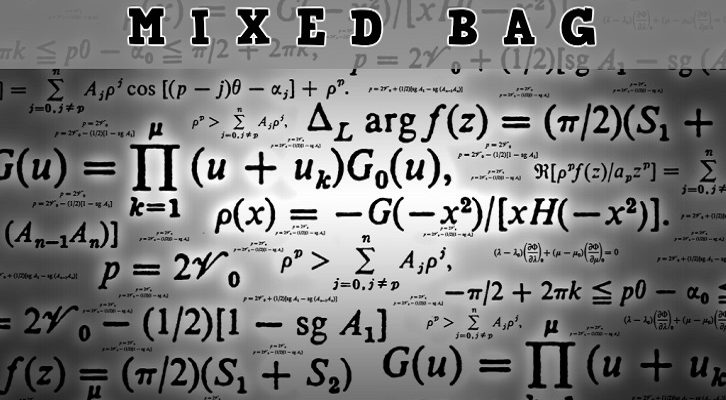# CAT – Logarithms

Question:
If log2X + log4X = log0.25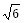and x > 0, then x is
A.  6-1/6
B.  61/6
C.  3-1/3
D.  61/3

Explanation:
log2x + log4x = log0.25log2x +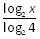= log0.25log2x *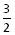= log0.25log2x * 3 = 2log0.25log2x3 = log0.256
log2x3 = -log46
log2x3 =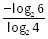log2x3 =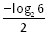2log2x3 = -log26
2log2x3 + log26 = 0
log26x6 = 0
6x6 = 1
x6 =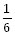x =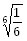Choice (A).
Level of difficulty 2

### Related posts

1.Durga swaroop Perla says:
2.ANANTHU R says:
3.ANANTHU R says: